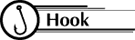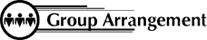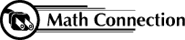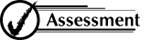8th Grade Math Home > Teacher Resources > Activities > View Tubes: Student Worksheet

View Tubes: Student Worksheet

Name: ____________________________________Does the length of a viewing tube, its diameter, or the distance from an object affect the type of data collected and the resulting graph?Work in groups of four or five.INVESTIGATION A

1. Collect the Data: Each member of the group will view the poster with the given tubes at varying distances from the wall. Others will mark the top and bottom of the described portion to determine the measurement in inches of the viewing height. Calculate the average visible height for each of the distances.
2. Graph the Data: Choose appropriate labels and scales for the horizontal and vertical axes. Plot the data as ordered pairs, (x, y).
3. Read the Results: Looking at your points, do they seem to lie along a line or a curve? Draw the line that best fits your data.
4. Describe in words how to determine the height of the visible portion if you know the distance from the wall.
5. Describe by equation how to determine the height of the visible portion (y) if you know the distance from the wall (x).
y =
6. Predict the height of the visible portion, if you were standing 10 feet
from the wall.
7. Predict the distance from the wall you would have to stand in order to see a 25-inch portion of the poster.

Viewing Height:
Same Tube Length & Tube Diameter
Varying Distances from Wall

 Student name Distance from Poster 1 foot 2 feet 3 feet 4 feet 5 feet 6 feet 7 feet 8 feet Total

INVESTIGATION B
1. Collect the Data: Each member of the group will view the poster with the given tubes at varying distances from the wall. Others will mark the top and bottom of the described portion to determine the measurement in inches of the viewing height. Calculate the average visible height for each of the distances.
2. Graph the Data: Choose appropriate labels and scales for the horizontal and vertical axes. Plot the data as ordered pairs, (x, y).
3. Read the Results: Looking at your points, do they seem to lie along a line or a curve? Draw the line that best fits your data.
4. Describe in words how to determine the height of the visible portion if you know the diameter of the tube.
5. Describe by equation how to determine the height of the visible portion (y) if you know the diameter of the tube (x).
y =
6. Predict the height of the visible portion for a tube with a diameter of five inches.
7. Predict the diameter of a tube that would allow you to see a 10-inch portion of the poster.

Viewing Height:
Same Distance from Wall & Tube Length
Varying Diameters

 Student Name Diameter of Tube 1" 2" 3" 4" 5" 6" Average

INVESTIGATION C
1. Collect the Data: Each member of the group will view the poster with the given tubes varying in length, a designated distance from the wall. Others will mark the top and bottom of the described portion to determine the measurement in inches of the viewing height. Calculate the average visible height for each of the lengths.
2. Graph the Data: Choose appropriate labels and scales for the horizontal and vertical axes. Plot the data as ordered pairs, (x, y).
3. Read the Results: Looking at your points, as the length of the tube increases, what is happening to the height of the visible portion of the picture?
4. Would the height of the portion ever decrease to zero? Why?
5. What height does the line seem to be approaching as a minimum?

Viewing Height
Same Tube Diameter & Distance from Wall
Varying Length

 Student Name Length of Tube 4 inches 7 inches 22 inches 33 inches AverageAs a result of this activity, students learn to analyze data that they have collected, look for relationships, and make predictions.Have students answer the following questions:

1. Explain how you knew the graphs were or were not linear.
2. Predict the height of the visible portion of the measuring tape if you were standing 15 feet from the wall.
3. Predict the distance you would have to stand from the wall in order to see a 30-inch portion of the measuring tape.
4. Predict the height of the visible portion for the tube with a diameter of 7.5 inches.
5. Predict the diameter of a tube that would allow you to see a 20-inch portion of the measuring tape.

 8th Grade Math Home > Teacher Resources > Activities > View Tubes: Student Worksheet